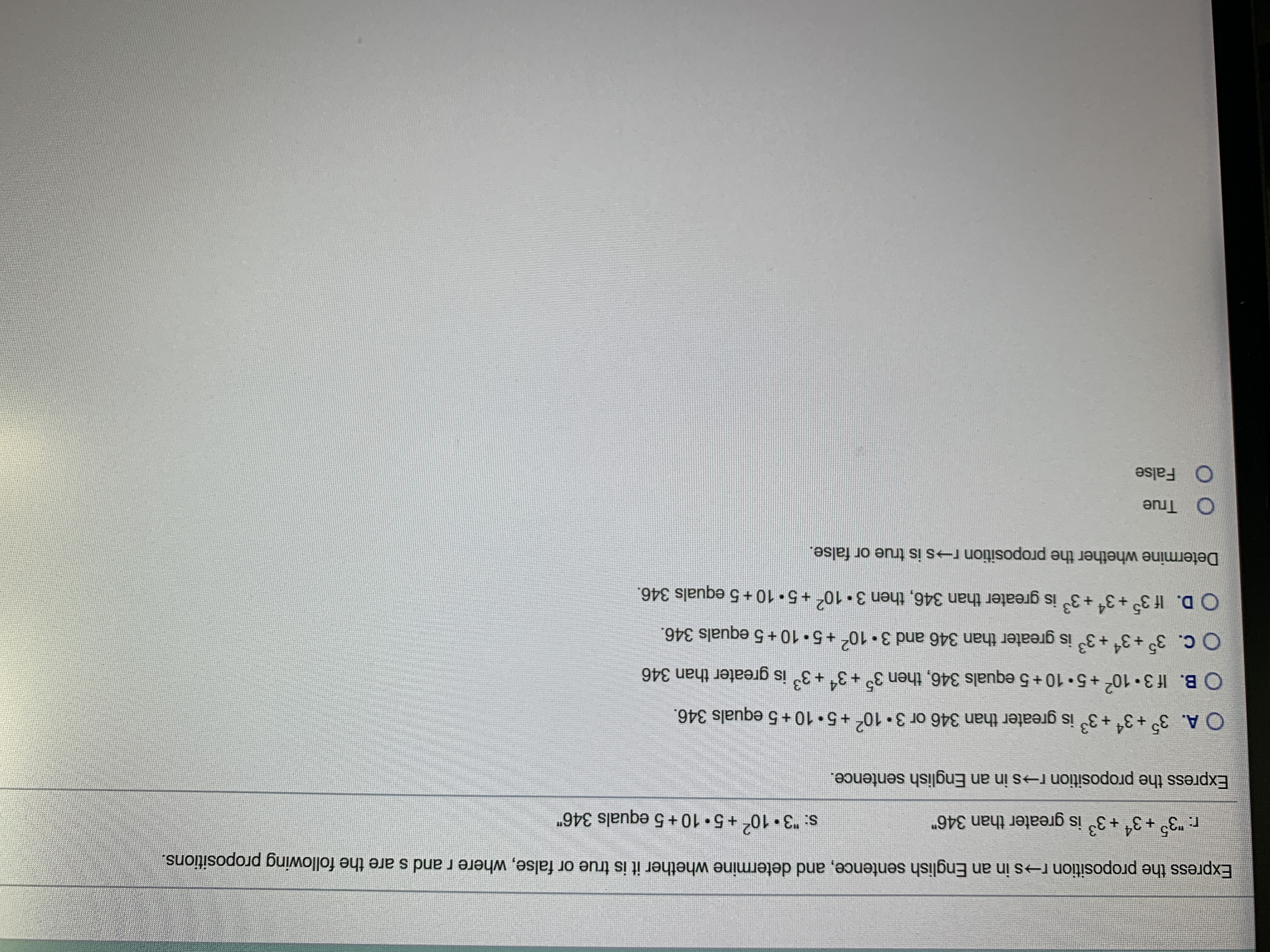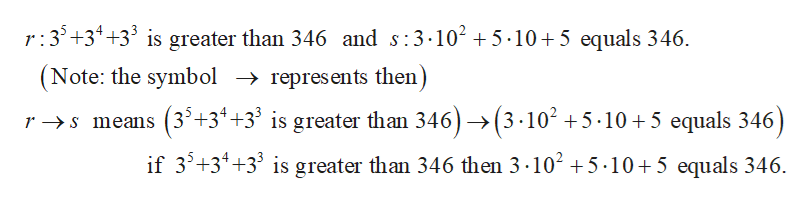# Express the proposition r→s in an English sentence, and determine whether it is true or false, where r and s are the following propositions.r. "3° +3 +3 is greater than 346"s: "3 10+5. 10 +5 equals 346"Express the proposition r→s in an English sentence.O A. 3° +3* +3° is greater than 346 or 310ʻ +5• 10 +5 equals 346.O B. If 3• 10 +5•10+5 equals 346, then 3° +3* +3° is greater than 346O C. 3° +3* +3° is greater than 346 and 3. 10+5 10 + 5 equals 346.O D. If 3° +3* +3° is greater than 346, then 3 10o +5• 10 + 5 equals 346.Determine whether the proposition r→s is true or false.O TrueO False

Question
56 viewshelp_outlineImage TranscriptioncloseExpress the proposition r→s in an English sentence, and determine whether it is true or false, where r and s are the following propositions. r. "3° +3 +3 is greater than 346" s: "3 10 +5. 10 +5 equals 346" Express the proposition r→s in an English sentence. O A. 3° +3* +3° is greater than 346 or 3 10ʻ +5• 10 +5 equals 346. O B. If 3• 10 +5•10+5 equals 346, then 3° +3* +3° is greater than 346 O C. 3° +3* +3° is greater than 346 and 3. 10 +5 10 + 5 equals 346. O D. If 3° +3* +3° is greater than 346, then 3 10o +5• 10 + 5 equals 346. Determine whether the proposition r→s is true or false. O True O False fullscreen
check_circle

Step 1help_outlineImage Transcriptioncloser:3'+3* +3° is greater than 346 and s:3.102 + 5 ·10+5 equals 346. (Note: the symbol → represents then) r → s means (3³+3* +3³ is greater than 346) → (3 ·10² + 5 ·10 + 5 equals 346) if 3'+3*+3' is greater than 346 then 3 ·102 +5 ·10+5 equals 346. fullscreen

### Want to see the full answer?

See Solution

#### Want to see this answer and more?

Solutions are written by subject experts who are available 24/7. Questions are typically answered within 1 hour.*

See Solution
*Response times may vary by subject and question.
Tagged in

### Math